search
Search
menu search toc more_vert
Guest 0reps
Thanks for the thanks!
close
Cancel
Post
account_circle
Profile
exit_to_app
Sign out
help Ask a question
search
keyboard_voice
close
Searching Tips
Search for a recipe:
"Creating a table in MySQL"
Search for an API documentation: "@append"
Search for code: "!dataframe"
Apply a tag filter: "#python"
Useful Shortcuts
/ to open search panel
Esc to close search panel
to navigate between search results
d to clear all current filters
Enter to expand content previewDoc SearchCode Search BetaSORRY NOTHING FOUND!
mic
Start speaking...Voice search is only supported in Safari and Chrome.
Shrink
Navigate to
A
A
brightness_medium
share
arrow_backShareTwitterFacebook
0
thumb_down
0
chat_bubble_outline
0
auto_stories new
settings

# Comprehensive guide on using Google Colab with Google Sheets

Software Development
chevron_right
schedule Sep 6, 2022
Last updated
local_offer
Tags

# Why use Google Colab with Google Sheets?

Google Colab is a free interactive notebook that allows you to run Python code on Google's virtual machines. One of the key selling points of Google Colab is that we can leverage Google's powerful GPU-equipped machines that come with 13GB of memory and over 100GB of disk space all without paying a penny. This means that Google Colab is more capable of manipulating and analyzing large amounts of data compared to Google Sheets!

On top of this, Google Colab comes with pre-installed data-related libraries like `pandas` and `scikit-learn`. Pandas allow you to manipulate your data much faster and more flexibly than in Google Sheets - check out our guide on getting started on Pandas for a quick introduction. Google Colab also allows you to build powerful machine learning models with libraries such as `scikit-learn` and `tensorflow` to make predictions and derive insights.

# Connecting Google Colab with Google Sheets

Before connecting Google Colab with Google Sheets, we must first give access to Google Drive on Colab using `auth.authenticate_user()`:

``` from google.colab import authauth.authenticate_user() ```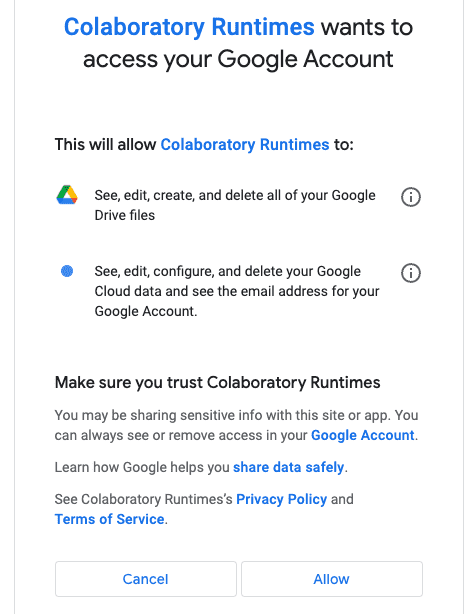We then connect and authenticate with Google sheets using the pre-installed `gspread` module:

``` from google.auth import defaultimport gspreadcreds, _ = default()gc = gspread.authorize(creds) ```

We can now proceed with interacting with the data in Google Sheets using Google Colab.

# Importing data from Google Sheets to Google Colab

Suppose we have a Google Sheet called `sample` in the root directory: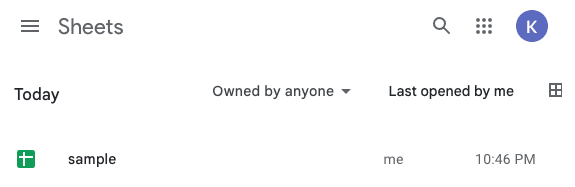The content of the `sample` file is as follows: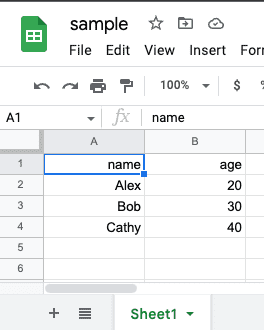To get the data inside this Google Sheet:

``` worksheet = gc.open('sample').sheet1list_of_dicts = worksheet.get_all_records()print(list_of_dicts) [{'name': 'Alex', 'age': 20}, {'name': 'Bob', 'age': 30}, {'name': 'Cathy', 'age': 40}] ```

Here, note the following:

• we can open up this Google Sheet using the `open('sample')` function

• we can access the first sheet using the property `sheet1`

• the `get_all_records()` method returns a list of dictionaries containing all the data stored in this sheet

You could also fetch the data as a list of lists using the `get_all_values(~)` method:

``` print(worksheet.get_all_values()) [['name', 'age'], ['Alex', '20'], ['Bob', '30'], ['Cathy', '40']] ```

## Reading the data stored in Google Sheets as a Pandas DataFrame

To read the data stored in Google Sheets as a Pandas DataFrame, simply pass the list of dictionaries returned by `get_all_records()` to the DataFrame constructor:

``` import pandas as pddf = pd.DataFrame(list_of_dicts)df.head() name age0 Alex 201 Bob 302 Cathy 40 ```

## Reading data using cell range

Suppose we wanted to fetch the values in range `A1:B3`: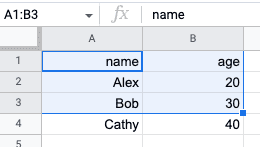We can use the `get(~)` method of a worksheet:

``` worksheet = gc.open('sample').sheet1worksheet.get('A1:B3') [['name', 'age'], ['Alex', '20'], ['Bob', '30']] ```

## Reading a specific row

To get the 2nd row, call `row_value(2)`:

``` worksheet = gc.open('sample').sheet1worksheet.row_values(2) ['Alex', '20'] ```

## Reading a specific column

To get the 2nd column, call `col_values(2)`:

``` worksheet = gc.open('sample').sheet1worksheet.col_values(2) ['age', '20', '30', '40'] ```

# Exporting data to Google Sheets from Google Colab

In most cases, the format in which you deal with data on Google Colab will be a Pandas DataFrame. If it is not (e.g. a list of numbers), then I highly recommend converting that into a DataFrame first.

Consider the following Pandas DataFrame:

``` df = pd.DataFrame({'A':[3,4],'B':[5,6]})df.head() A B0 3 51 4 6 ```

The first step in exporting this DataFrame into Google Sheets is to convert the DataFrame into a list of lists:

``` values = [df.columns.values.tolist()] + df.values.tolist()values [['A', 'B'], [3, 5], [4, 6]] ```

Here, the first list contains the column labels while the rest are the values of the DataFrame.

Next, we create a new Google Sheets file using the `create(~)` function, which returns a `GoogleSheets `object. We then access the `sheet1` property, and then perform the update:

``` sheet = gc.create('new_sheet').sheet1sheet.update(values) {'spreadsheetId': '1otc5DNi-6EPBbTAoaKwrwCJV5X78iH8ZEa1KdDLpgyA', 'updatedCells': 6, 'updatedColumns': 2, 'updatedRange': 'Sheet1!A1:B3', 'updatedRows': 3} ```

Here, we can see that the `update(~)` method has returned a dictionary containing some information about how the sheet was populated.

WARNING

If a Google Sheet with the same name exists, then the `create(~)` method will overwrite that file.

After running this code, we should see our newly created Google Sheets: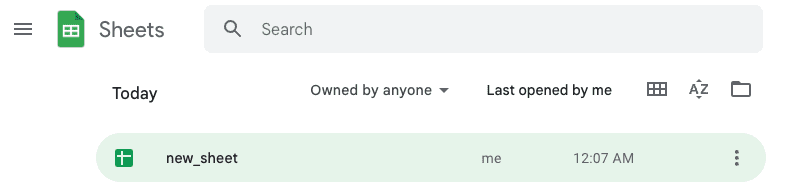Opening this file reveals the following: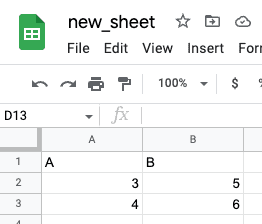For your convenience, here's a function that combines our code snippets in one single operation:

``` def write_df_to_sheets(df, sheet_name): values = [df.columns.values.tolist()] + df.values.tolist() sheet = gc.create(sheet_name).sheet1 sheet.update(values) ```

We call this method like so:

``` df = pd.DataFrame({'A':[3,4],'B':[5,6]})write_df_to_sheets(df, 'new_sheet') ```

## Appending new rows to Google Sheets

Suppose our Google Sheet called `sample` was as follows: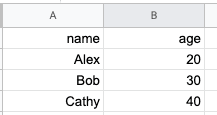We can append new rows by using the worksheet's `append_row(~)` method:

``` worksheet = gc.open('sample').sheet1worksheet.append_rows([['David', 60], ['Eric', 70]]) {'spreadsheetId': '1Myb733ychkWFNipd75YFX6glZTE0o0Sgx-yaUpz58G8', 'tableRange': 'Sheet1!A1:B4', 'updates': {'spreadsheetId': '1Myb733ychkWFNipd75YFX6glZTE0o0Sgx-yaUpz58G8', 'updatedCells': 4, 'updatedColumns': 2, 'updatedRange': 'Sheet1!A5:B6', 'updatedRows': 2}} ```

Running this code would give us the following updated Google Sheet: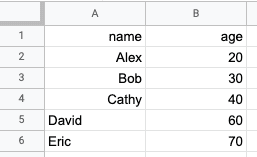# General workflow of using the gspread library

The `gspread` library comes with methods that allow you to manipulate data on Google Sheets (e.g. `append_rows(~)` for appending new rows). However, the methods are limited and you'll find that in most cases there is no built-in method to achieve what you're trying to do. Therefore, the general workflow is to read the data in Google Sheets using a Pandas DataFrame, and then perform data manipulation using Pandas.

As an example, suppose we have the following Google Sheets called `sample`:Now suppose we wanted to add a new column called `status`, which is a categorical column holding the values `'JUNIOR'` or `'SENIOR'` based on the `age` value. We cannot do this using the `gspread` library, so we need to use Pandas.

Once again, to import the data in Google Sheets as a Pandas DataFrame:

``` import pandas as pdworksheet = gc.open('sample').sheet1list_of_dicts = worksheet.get_all_records()df = pd.DataFrame(list_of_dicts)df.head() name age0 Alex 201 Bob 302 Cathy 40 ```

Let's append the new column `status` using NumPy's `where(~)` method:

``` import numpy as npdf['status'] = np.where(df['age'] < 25, 'JUNIOR', 'SENIOR')df.head() name age status0 Alex 20 JUNIOR1 Bob 30 SENIOR2 Cathy 40 SENIOR ```

Finally, let's call our method `write_df_to_sheets(~)` that we defined earlier:

``` write_df_to_sheets(df, 'new_sheet') ```

We should then see a new Google sheet called `new_sheet` with the appended `status` column: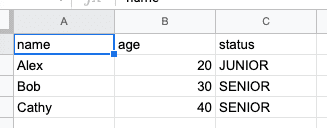mail